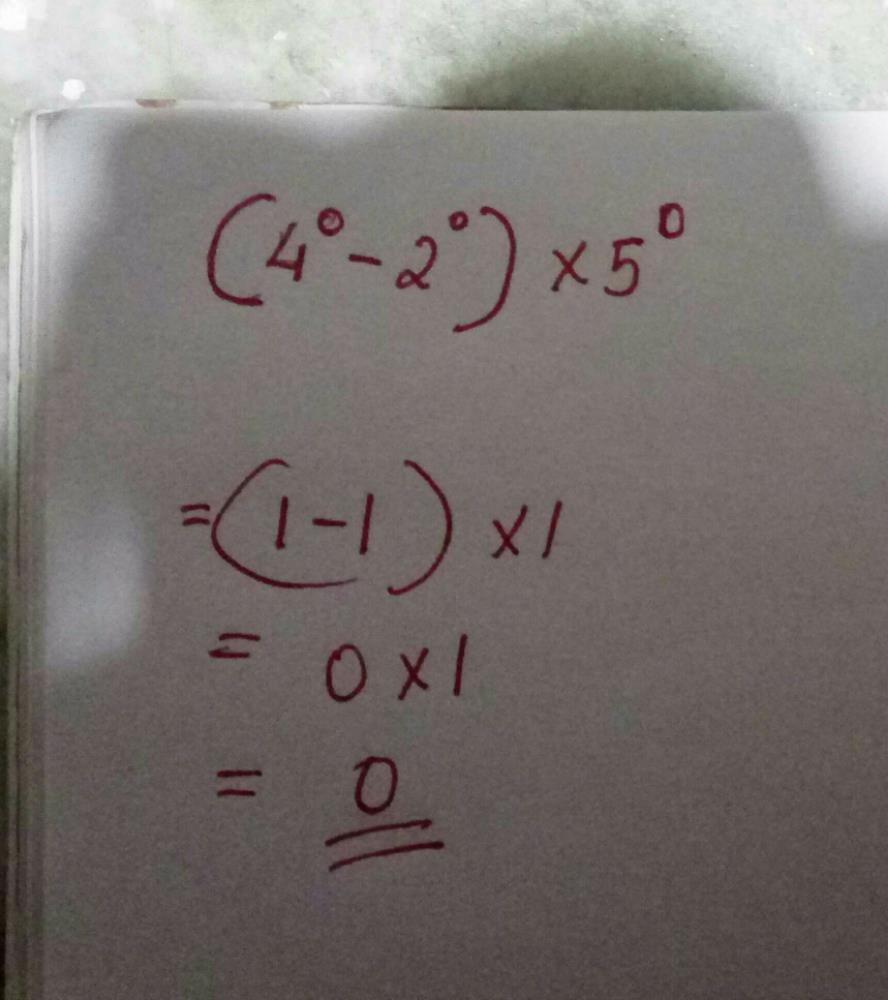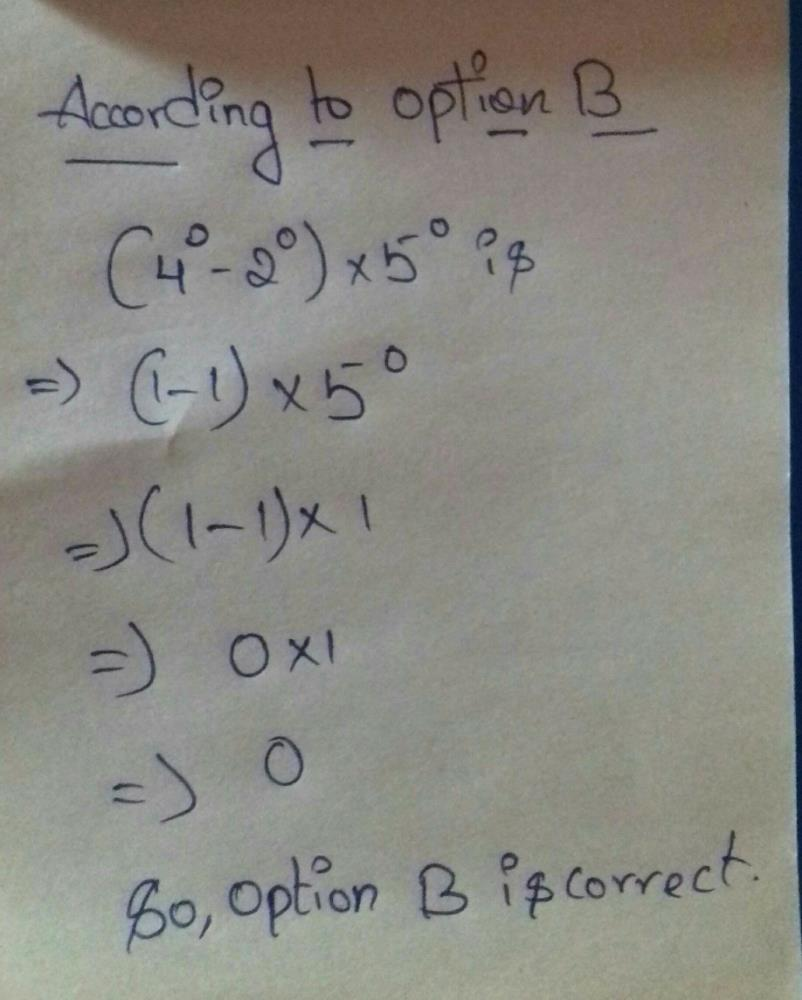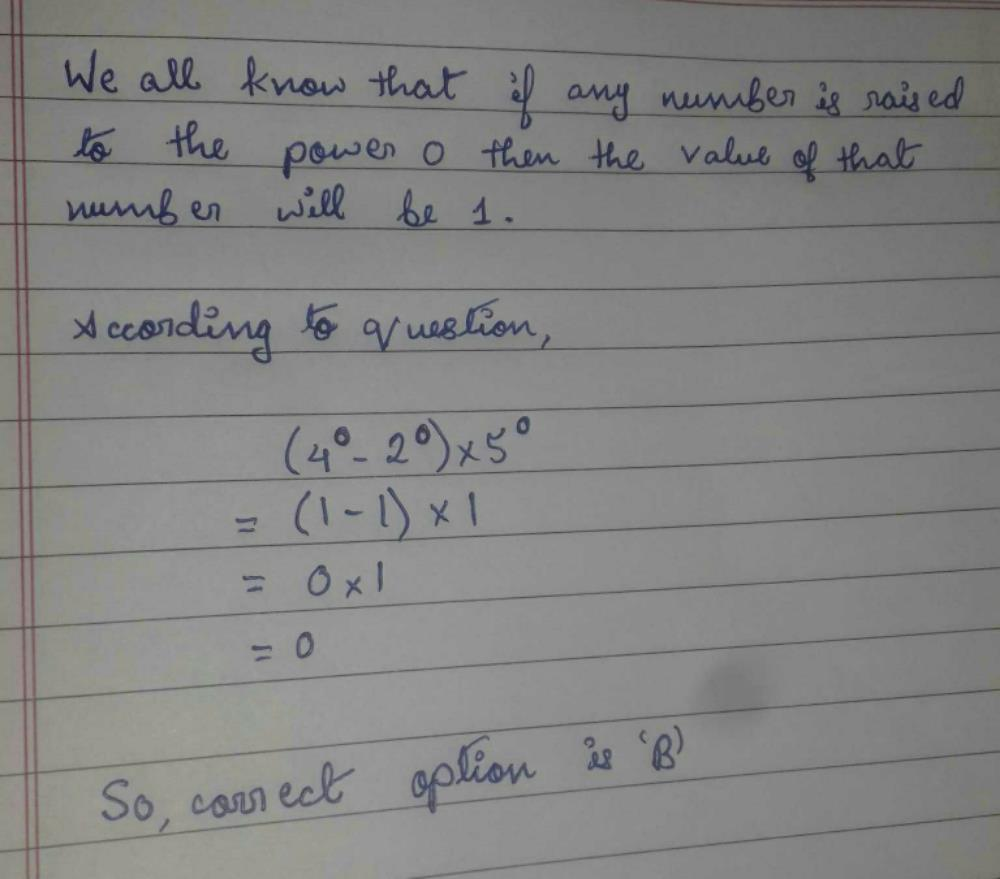Courses

# The value of (40 - 20) x 50 isa)1b)0c)10d)-10Correct answer is option 'B'. Can you explain this answer?

## Class 7 Question

By Karanjot Singh · Dec 30, 2019 ·Class 7
Aishwarya Sreesh answered 5 days agoSuribabu Mallavarapu answered 4 weeks agoKushum Lahary answered Dec 30, 2019Suman Verma answered Dec 30, 2019
But in phone it was written as (40 - 20)50??? what is this

Satvik Kumar answered Dec 31, 2019
Yes option but because as we know that any number with power 0 =1 then the equation =[1-1]×1=0×1=0

Tanshmeet Kaur answered Aug 31, 2019
Anything raised to the power zero is 1 .
In the given expression,  (4- 20) x 50
= (1 - 1) x 1
= ( 0 ) x 1
= 0
So Option B is correct answer.

Santosh Bara answered 1 week ago
Answer Is ten why zero value is one

Naresh Patel answered 3 weeks ago
(40-20)×50
(1-1)×1. (a0=1)
0×1
0

Jyotsna Kshirsagar answered Jan 29, 2020
B